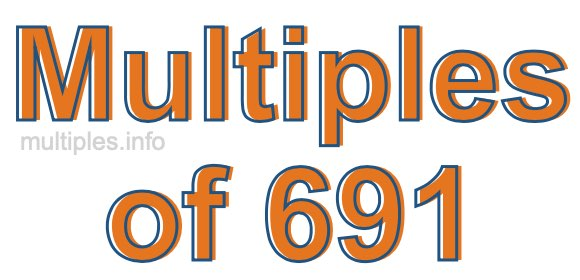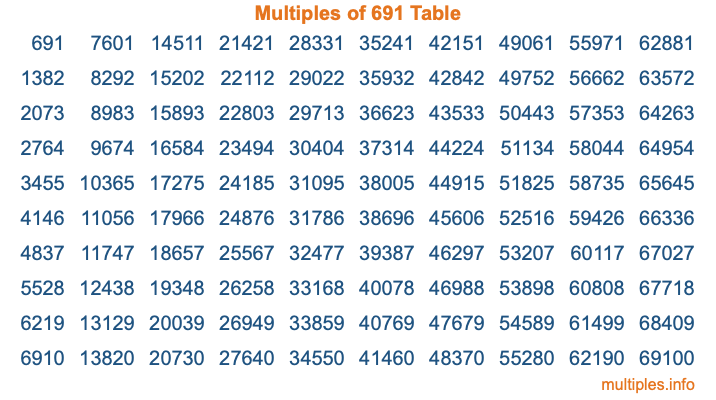Multiples of 691Welcome to the Multiples of 691 page. Here we will first teach you everything you will ever need to know about the multiples of 691, and then give you a study guide summary of everything we taught you to make sure you remember it all. Use this page to look up facts and learn information about the multiples of 691. This page will make you a multiples of six hundred ninety-one expert!

Definition of Multiples of 691
Multiples of 691 are all the numbers that when divided by 691 equal an integer. Each of the multiples of 691 are called a multiple. A multiple of 691 is created by multiplying 691 by an integer.

Therefore, to create a list of multiples of 691, you start with 1 multiplied by 691, then 2 multiplied by 691, then 3 multiplied by 691, and so on for as long as you want. Thus, the list of the first five multiples of 691 is 691, 1382, 2073, 2764, and 3455. To see a larger list of multiples of 691, see the printable image of Multiples of 691 further down on this page. We also have a category where you can choose any nth multiple of 691.

Multiples of 691 Checker
The Multiples of 691 Checker below checks to see if any number of your choice is a multiple of 691. In other words, it checks to see if there is any number (integer) that when multiplied by 691 will equal your number. To do that, we divide your number by 691. If the the quotient is an integer, then your number is a multiple of 691.

Is  a multiple of 691?

Least Common Multiple of 691 and ...
A Least Common Multiple (LCM) is the lowest multiple that two or more numbers have in common. This is also called the smallest common multiple or lowest common multiple and is useful to know when you are adding our subtracting fractions. Enter one or more numbers below (691 is already entered) to find the LCM.

Check out our LCM Calculator if you need more details about the Least Common Multiple or if you need the LCM for different numbers for adding and subtraction fractions.

nth Multiple of 691
As we stated above, 691 is the first multiple of 691, 1382 is the second multiple of 691, 2073 is the third multiple of 691, and so on. Enter a number below to find the nth multiple of 691.

th multiple of 691

Multiples of 691 vs Factors of 691
691 is a multiple of 691 and a factor of 691, but that is where the similarities end. All postive multiples of 691 are 691 or greater than 691. All positive factors of 691 are 691 or less than 691.

Below is the beginning list of multiples of 691 and the factors of 691 so you can compare:

Multiples of 691: 691, 1382, 2073, 2764, 3455, etc.

Factors of 691: 1, 691

As you can see, the multiples of 691 are all the numbers that you can divide by 691 to get a whole number. The factors of 691, on the other hand, are all the whole numbers that you can multiply by another whole number to get 691.

It's also interesting to note that if a number (x) is a factor of 691, then 691 will also be a multiple of that number (x).

Multiples of 691 vs Divisors of 691
The divisors of 691 are all the integers that 691 can be divided by evenly. Below is a list of the divisors of 691.

Divisors of 691: 1, 691

The interesting thing to note here is that if you take any multiple of 691 and divide it by a divisor of 691, you will see that the quotient is an integer.

Multiples of 691 Table
Below is an image of the first 100 multiples of 691 in a table. The table is in chronological order, column by column. The first column has the first ten multiples of 691, the second column has the next ten multiples of 691, and so on.The Multiples of 691 Table is also referred to as the 691 Times Table or Times Table of 691. You are welcome to print out our table for your studies.

Negative Multiples of 691
Although not often discussed or needed in math, it is worth mentioning that you can make a list of negative multiples of 691 by multiplying 691 by -1, then by -2, then by -3, and so on, to get the following list of negative multiples of 691:

-691, -1382, -2073, -2764, -3455, etc.

Multiples of 691 Summary
Below is a summary of important Multiples of 691 facts that we have discussed on this page. To retain the knowledge on this page, we recommend that you read through the summary and explain to yourself or a study partner why they hold true.

There are an infinite number of multiples of 691.

A multiple of 691 divided by 691 will equal a whole number.

691 divided by a factor of 691 equals a divisor of 691.

The nth multiple of 691 is n times 691.

The largest factor of 691 is equal to the first positive multiple of 691.

691 is a multiple of every factor of 691.

691 is a multiple of 691.

A multiple of 691 divided by a divisor of 691 equals an integer.

691 divided by a divisor of 691 equals a factor of 691.

Any integer times 691 will equal a multiple of 691.

Multiples of a Number
Here you can get the multiples of another number, all with the same attention to detail as we did for multiples of 691 on this page.

Multiples of
Multiples of 692
Did you find our page about multiples of six hundred ninety-one educational? Do you want more knowledge? Check out the multiples of the next number on our list!